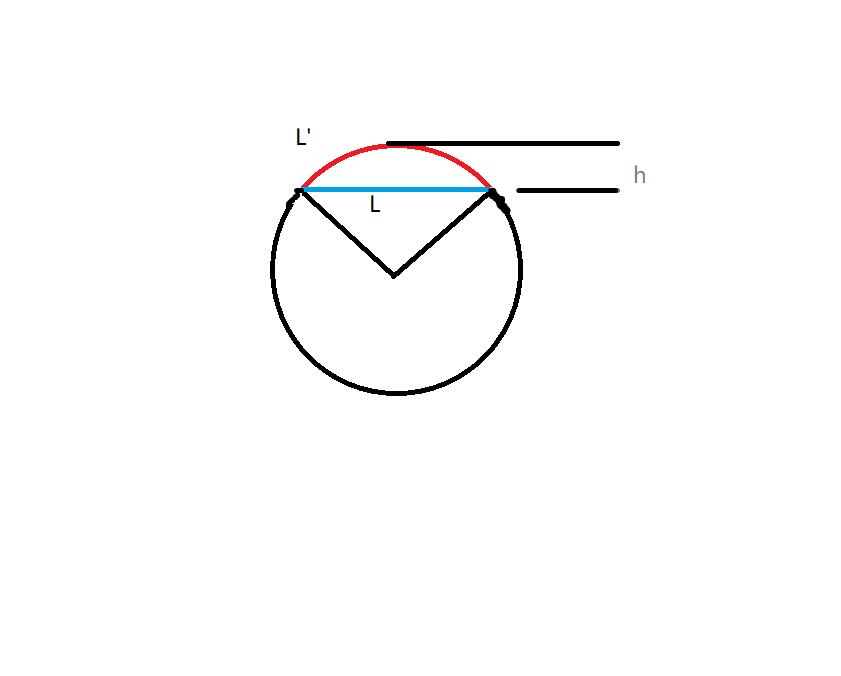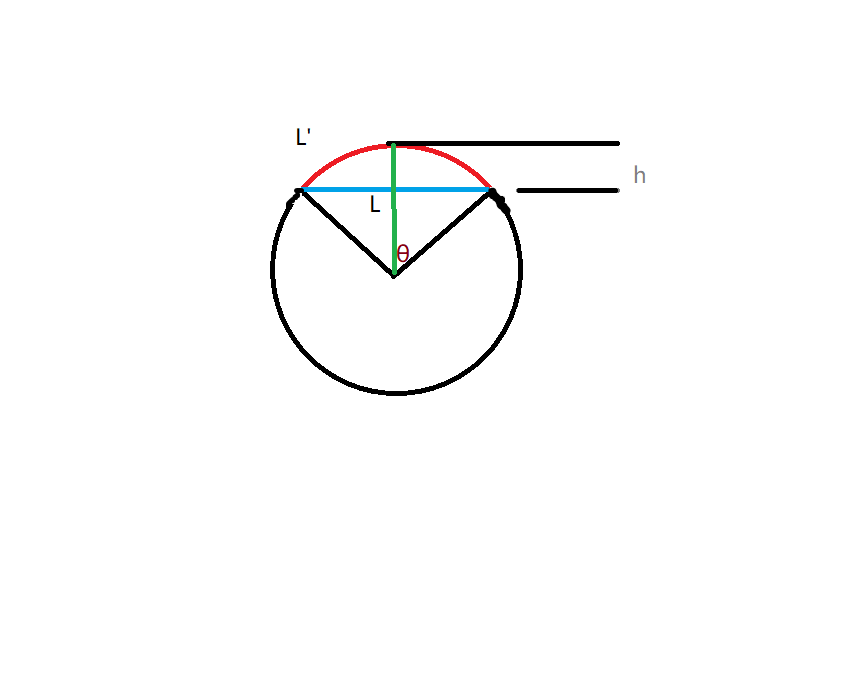## POJ-1905

When a thin rod of length L is heated n degrees, it expands to a new length L’=(1+nC)L, where C is the coefficient of heat expansion.
When a thin rod is mounted on two solid walls and then heated, it expands and takes the shape of a circular segment, the original rod being the chord of the segment.
Your task is to compute the distance by which the center of the rod is displaced.θ=arcsin(L/2R)
L’=2θ/(2π) * 2πR=2Rθ
L=2sqrt((R * R) -(R-h) * (R-h))

L’=2Rarcsin(L/2R) ①
R=(L * L+4h
h)/(8h) ②

s= L’=(4
hh+LL)/4.0/hasin(4hL/(4h*+L*L))
s对h求导得到s’在h>=0时是单调增加的

``````#include <cstdio>
#include <cmath>
#include <cstdlib>
#include <iostream>
#include <iomanip>
using namespace std;
const double esp=1e-5;
int main()
{
while (true)
{
double L,n,c,ans=0,s;
cin>>L>>n>>c;
if (L<0) break;
s=(1+n*c)*L;
double l=0.0,r=L;
while (l+esp<=r)
{
double mid=(l+r)/2;
double R=(4*mid*mid+L*L)/(8*mid);
double a=2*R*asin(L/(2*R));
if (a<=s) l=mid;
else r=mid;
ans=mid;
}
printf("%.3f\n",ans);
}
}
``````